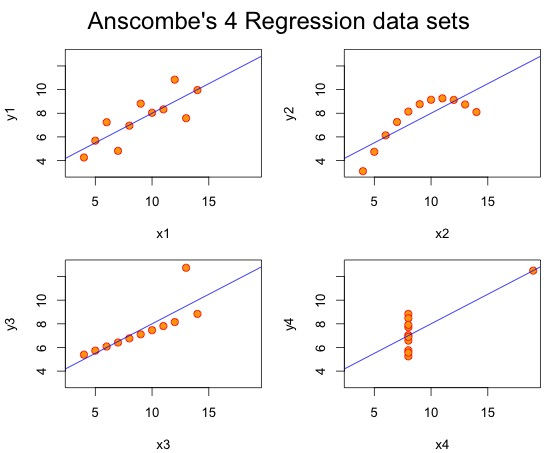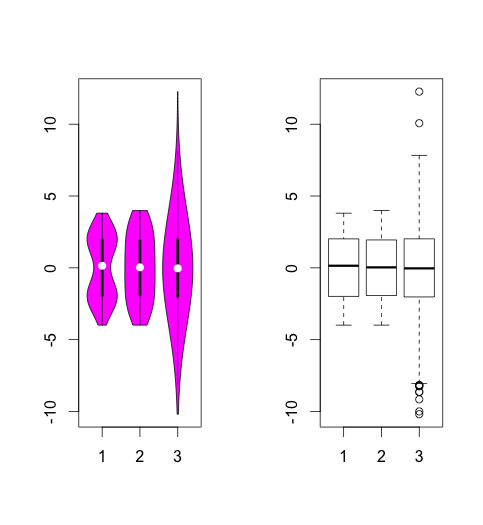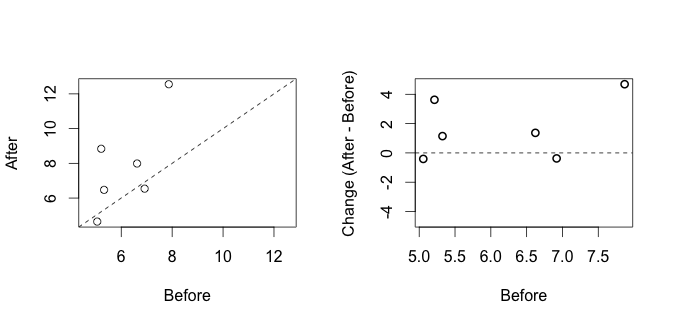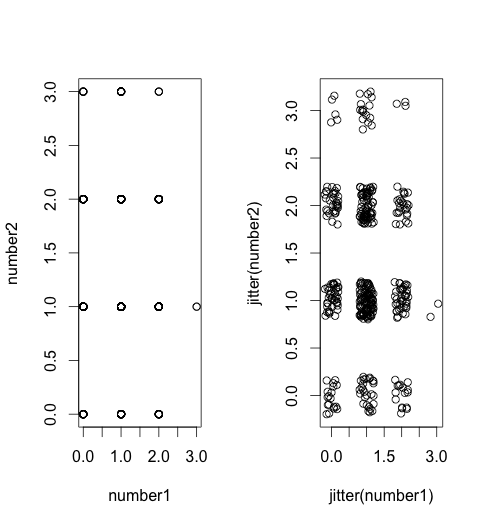1037 字

# 另一个安斯库姆四重奏？Plos Biology 上刚发表了一篇PERSPECTIVE来吐槽条形图，作者收集了2014年一季度前25%的生理学期刊中发表的703篇文章并对其中的图像进行了统计。结果如下：

• 条形图是这些文章作者最喜欢的图形展示方法，85.6%的文章包含至少一张条形图
• 77.6%的条形图使用的是均值配合标准误，15.3%使用均值配合标准差
• 61.3%的文章使用线图，散点图配合误差线，误差多数使用标准误
• 使用直方图，散点图与箱式图等展示数据分布的不到五分之一

• 你们懂不懂啥时候用SD啥时候用SE啊
• 不懂没关系啊，反正都不该用啊
• 那些都是描述性统计啊，你样本那么少直接画出来多好，算什么均值啊
• 展示数据跟假设检验两个概念，别混了啊
• 做对比用t检验你查过数据分布了吗？有异常值检验啊
• 不会没关系啊，反正我建议用非参方法啊，当然我知道损失功效啊
• 用非参方法要展示中值啊，中值的差跟差的中值不是一回事啊• 小提琴图
``````par(mfrow=c(1,2))
mu<-2
si<-0.6
bimodal<-c(rnorm(1000,-mu,si),rnorm(1000,mu,si))
uniform<-runif(2000,-4,4)
normal<-rnorm(2000,0,3)
vioplot(bimodal,uniform,normal)
boxplot(bimodal,uniform,normal)
``````• 差异散点图
``````# 这个太简单了，就是当要展示的两组数据为配对数据时，直接对其差异作普通散点图并附上参考线
``````• 抖动散点图
``````number1 <- rhyper(400,3,6,3)
number2 <- rhyper(400,4,5,3)
par(mfrow=c(1,2))
plot(number1,number2)
plot(jitter(number1),jitter(number2))
``````• 平滑散点图
``````par(mfrow=c(1,1))
number1 <- rhyper(30,4,5,4)
number2 <- rhyper(30,4,5,4)
smoothScatter(number1,number2)
``````## 参考文献

Beyond Bar and Line Graphs: Time for a New Data Presentation Paradigm（开放获取）